# Shear and moment diagram

1 de Nov de 2013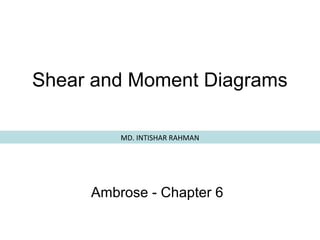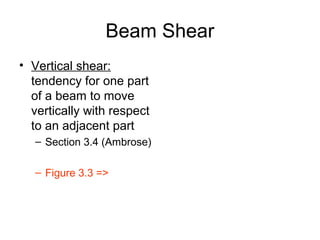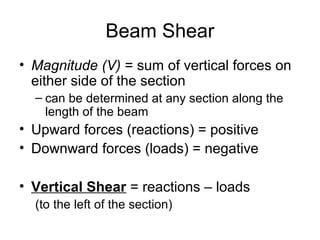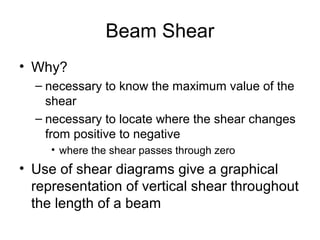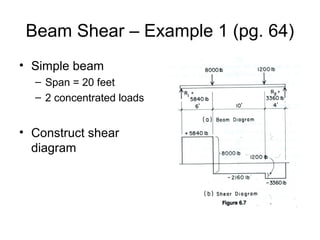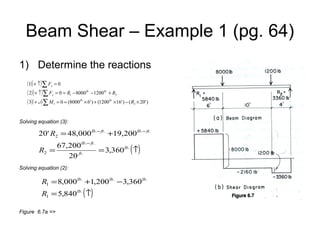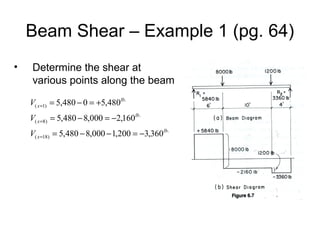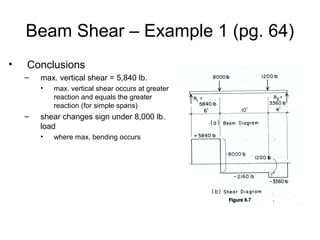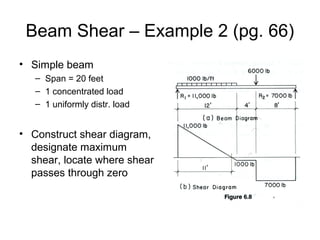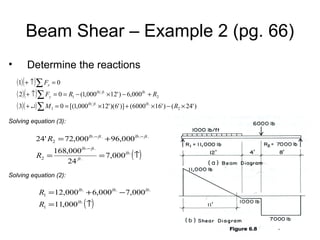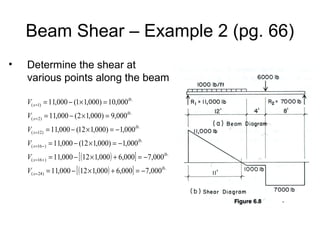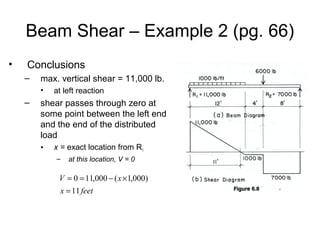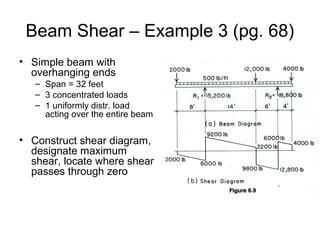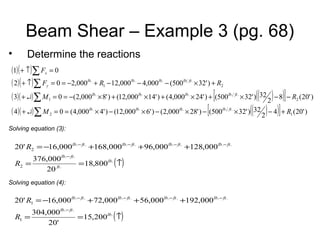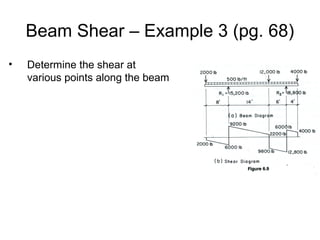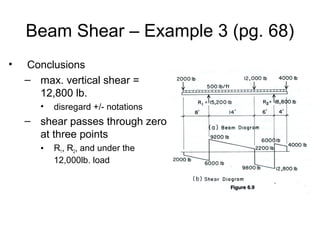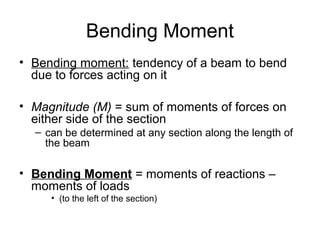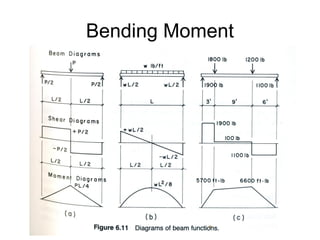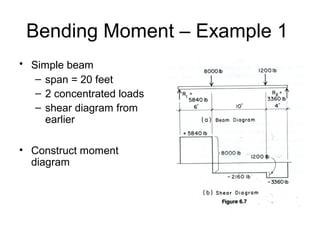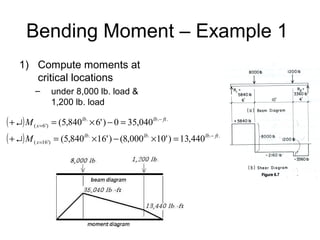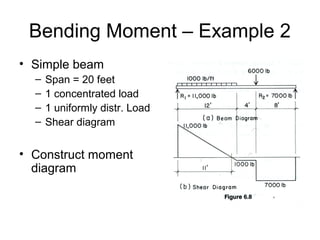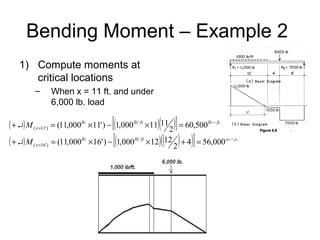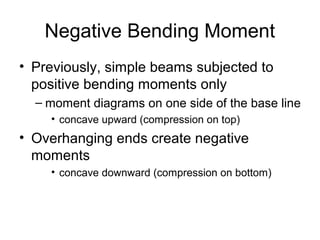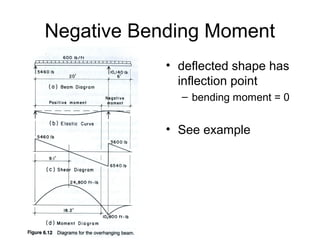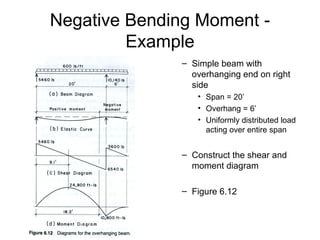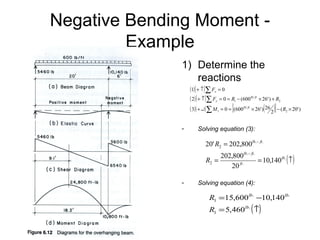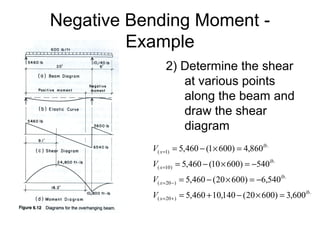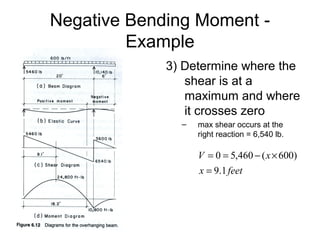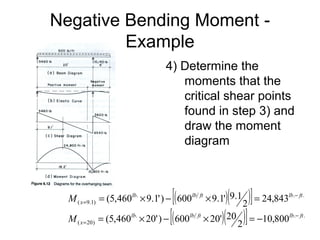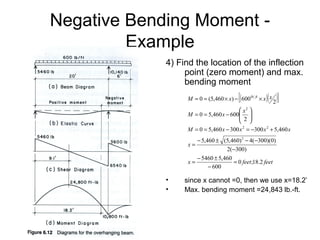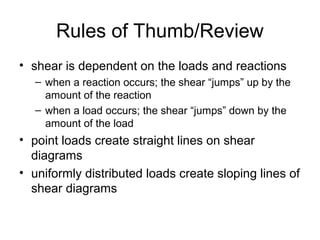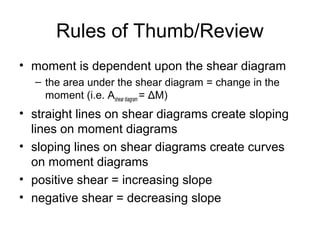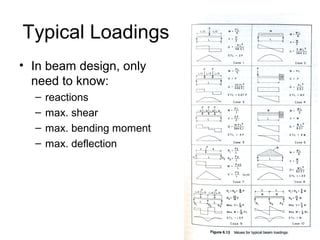1 de 33

### Shear and moment diagram

1. Shear and Moment Diagrams MD. INTISHAR RAHMAN Ambrose - Chapter 6
2. Beam Shear • Vertical shear: tendency for one part of a beam to move vertically with respect to an adjacent part – Section 3.4 (Ambrose) – Figure 3.3 =>
3. Beam Shear • Magnitude (V) = sum of vertical forces on either side of the section – can be determined at any section along the length of the beam • Upward forces (reactions) = positive • Downward forces (loads) = negative • Vertical Shear = reactions – loads (to the left of the section)
4. Beam Shear • Why? – necessary to know the maximum value of the shear – necessary to locate where the shear changes from positive to negative • where the shear passes through zero • Use of shear diagrams give a graphical representation of vertical shear throughout the length of a beam
5. Beam Shear – Example 1 (pg. 64) • Simple beam – Span = 20 feet – 2 concentrated loads • Construct shear diagram
6. Beam Shear – Example 1 (pg. 64) 1) Determine the reactions (1) ( + ↑ )∑ Fx = 0 ( 2) ( + ↑ )∑ Fy = 0 = R1 − 8000lb. − 1200lb. + R2 ( 3)( + ↵) ∑ M 1 = 0 = (8000lb. × 6' ) + (1200lb. ×16' ) − ( R2 × 20' ) Solving equation (3): 20' R2 = 48,000lb.− ft . +19,200 lb.− ft . 67,200lb.− ft . R2 = = 3,360lb. (↑) 20 ft . Solving equation (2): R1 = 8,000lb. +1,200lb. − 3,360lb. R1 = 5,840 lb. (↑) Figure 6.7a =>
7. Beam Shear – Example 1 (pg. 64) • Determine the shear at various points along the beam V( x =1) = 5,480 − 0 = +5,480lb. V( x =8) = 5,480 − 8,000 = −2,160lb. V( x =18) = 5,480 − 8,000 − 1,200 = −3,360lb.
8. Beam Shear – Example 1 (pg. 64) • Conclusions – max. vertical shear = 5,840 lb. • – max. vertical shear occurs at greater reaction and equals the greater reaction (for simple spans) shear changes sign under 8,000 lb. load • where max. bending occurs
9. Beam Shear – Example 2 (pg. 66) • Simple beam – Span = 20 feet – 1 concentrated load – 1 uniformly distr. load • Construct shear diagram, designate maximum shear, locate where shear passes through zero
10. Beam Shear – Example 2 (pg. 66) • Determine the reactions (1) ( + ↑)∑ Fx = 0 ( 2) ( + ↑)∑ Fy = 0 = R1 − (1,000lb ft. ×12' ) − 6,000lb. + R2 ( 3)( + ↵) ∑ M 1 = 0 = [(1,000lb ft. ×12' )(6' )] + (6000lb. ×16' ) − ( R2 × 24' ) Solving equation (3): 24' R2 = 72,000lb.− ft . + 96,000 lb.− ft . 168,000lb.− ft . R2 = = 7,000lb. (↑) 24 ft . Solving equation (2): R1 =12,000lb. + 6,000lb. − 7,000lb. R1 =11,000lb. (↑)
11. Beam Shear – Example 2 (pg. 66) • Determine the shear at various points along the beam V( x =1) = 11,000 − (1× 1,000) = 10,000lb. V( x = 2) = 11,000 − (2 × 1,000) = 9,000lb. V( x =12) = 11,000 − (12 × 1,000) = −1,000lb. V( x =16 − ) = 11,000 − (12 × 1,000) = −1,000lb. V( x =16 + ) = 11,000 − [ (12 × 1,000 ) + 6,000] = −7,000lb. V( x = 24 ) = 11,000 − [ (12 × 1,000) + 6,000] = − 7,000lb.
12. Beam Shear – Example 2 (pg. 66) • Conclusions – max. vertical shear = 11,000 lb. • – at left reaction shear passes through zero at some point between the left end and the end of the distributed load • x = exact location from R1 – at this location, V = 0 V = 0 = 11,000 − ( x ×1,000) x = 11 feet
13. Beam Shear – Example 3 (pg. 68) • Simple beam with overhanging ends – Span = 32 feet – 3 concentrated loads – 1 uniformly distr. load acting over the entire beam • Construct shear diagram, designate maximum shear, locate where shear passes through zero
14. Beam Shear – Example 3 (pg. 68) • Determine the reactions (1) ( + ↑)∑ Fx = 0 ( 2) ( + ↑)∑ Fy = 0 = −2,000lb. + R1 − 12,000lb. − 4,000lb. − (500lb ft. × 32' ) + R2 ( 3)( + ↵) ∑ M 1 = 0 = −(2,000lb. × 8' ) + (12,000lb. ×14' ) + (4,000lb. × 24' ) + [(500lb. ft . × 32' )((32 2 ) − 8)] − R2 (20' ) ( 4)( + ↵) ∑ M 2 = 0 = (4,000lb. × 4' ) − (12,000lb. × 6' ) − (2,000lb. × 28' ) − [(500lb. ft . × 32' )((32 2 ) − 4)] + R1 (20' ) Solving equation (3): 20' R2 = −16,000lb.− ft . +168,000lb.− ft . + 96,000lb.− ft . +128,000lb.− ft . 376,000lb.− ft . R2 = =18,800lb. (↑) ft . 20 Solving equation (4): 20' R1 = −16,000lb.− ft . + 72,000lb.− ft . + 56,000lb.− ft . +192,000lb.− ft . 304,000lb.− ft . R1 = =15,200lb. (↑) 20'
15. Beam Shear – Example 3 (pg. 68) • Determine the shear at various points along the beam
16. Beam Shear – Example 3 (pg. 68) • Conclusions – max. vertical shear = 12,800 lb. • disregard +/- notations – shear passes through zero at three points • R1, R2, and under the 12,000lb. load
17. Bending Moment • Bending moment: tendency of a beam to bend due to forces acting on it • Magnitude (M) = sum of moments of forces on either side of the section – can be determined at any section along the length of the beam • Bending Moment = moments of reactions – moments of loads • (to the left of the section)
18. Bending Moment
19. Bending Moment – Example 1 • Simple beam – span = 20 feet – 2 concentrated loads – shear diagram from earlier • Construct moment diagram
20. Bending Moment – Example 1 1) Compute moments at critical locations – under 8,000 lb. load & 1,200 lb. load ( + ↵) M ( x =6') = (5,840lb. × 6' ) − 0 = 35,040lb.− ft . ( + ↵) M ( x =16') = (5,840lb. ×16' ) − (8,000lb. ×10' ) = 13,440lb.− ft .
21. Bending Moment – Example 2 • Simple beam – – – – Span = 20 feet 1 concentrated load 1 uniformly distr. Load Shear diagram • Construct moment diagram
22. Bending Moment – Example 2 1) Compute moments at critical locations – When x = 11 ft. and under 6,000 lb. load ( + ↵) M ( x =11') = (11,000lb. ×11' ) − [(1,000lb ft ×11)(11 2 )] = 60,500lb.− ft . ( + ↵) M ( x =16') = (11,000lb. ×16' ) − [(1,000lb ft ×12)((12 2 ) + 4)] = 56,000lb.− ft .
23. Negative Bending Moment • Previously, simple beams subjected to positive bending moments only – moment diagrams on one side of the base line • concave upward (compression on top) • Overhanging ends create negative moments • concave downward (compression on bottom)
24. Negative Bending Moment • deflected shape has inflection point – bending moment = 0 • See example
25. Negative Bending Moment Example – Simple beam with overhanging end on right side • Span = 20’ • Overhang = 6’ • Uniformly distributed load acting over entire span – Construct the shear and moment diagram – Figure 6.12
26. Negative Bending Moment Example 1) Determine the reactions (1) ( + ↑)∑ Fx = 0 ( 2) ( + ↑)∑ Fy = 0 = R1 − (600lb ft. × 26' ) + R2 ( 3)( + ↵) ∑ M 1 = 0 = [(600lb ft. × 26' )(26 2 )] − ( R2 × 20' ) - Solving equation (3): 20' R2 = 202,800lb.− ft . 202,800lb.− ft . R2 = = 10,140lb. ( ↑ ) ft . 20 - Solving equation (4): R1 =15,600lb. −10,140lb. R1 = 5,460lb. (↑)
27. Negative Bending Moment Example 2) Determine the shear at various points along the beam and draw the shear diagram V( x =1) = 5,460 − (1× 600) = 4,860lb. V( x =10 ) = 5,460 − (10 × 600) = −540lb. V( x = 20 − ) = 5,460 − (20 × 600) = −6,540lb. V( x = 20 + ) = 5,460 + 10,140 − (20 × 600) = 3,600lb.
28. Negative Bending Moment Example 3) Determine where the shear is at a maximum and where it crosses zero – max shear occurs at the right reaction = 6,540 lb. V = 0 = 5,460 − ( x × 600) x = 9.1 feet
29. Negative Bending Moment Example 4) Determine the moments that the critical shear points found in step 3) and draw the moment diagram [( )] = 24,843 2 × 20') ( 20 )] = −10,800 2 )( M ( x =9.1) = (5,460lb. × 9.1' ) − 600lb ft × 9.1' 9.1 lb .− ft . M ( x = 20) = (5,460lb. × 20' ) − 600lb lb.− ft . [( ft
30. Negative Bending Moment Example 4) Find the location of the inflection point (zero moment) and max. bending moment [( ) ( 2 )] M = 0 = (5,460 × x) − 600lb ft × x x  x2  M = 0 = 5,460 x − 600   2   M = 0 = 5,460 x − 300 x 2 = −300 x 2 + 5,460 x − 5,460 ± (5,460) 2 − 4(−300)(0) x= 2(−300) − 5460 ± 5,460 x= = 0 feet;18.2 feet − 600 • • since x cannot =0, then we use x=18.2’ Max. bending moment =24,843 lb.-ft.
31. Rules of Thumb/Review • shear is dependent on the loads and reactions – when a reaction occurs; the shear “jumps” up by the amount of the reaction – when a load occurs; the shear “jumps” down by the amount of the load • point loads create straight lines on shear diagrams • uniformly distributed loads create sloping lines of shear diagrams
32. Rules of Thumb/Review • moment is dependent upon the shear diagram – the area under the shear diagram = change in the moment (i.e. Ashear diagram = ΔM) • straight lines on shear diagrams create sloping lines on moment diagrams • sloping lines on shear diagrams create curves on moment diagrams • positive shear = increasing slope • negative shear = decreasing slope
33. Typical Loadings • In beam design, only need to know: – – – – reactions max. shear max. bending moment max. deflection